# Volume - Definition with Examples

The Complete K-5 Math Learning Program Built for Your Child

• 40 Million Kids

Loved by kids and parent worldwide

• 50,000 Schools

Trusted by teachers across schools

• Comprehensive Curriculum

Aligned to Common Core

##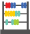Let's learn!

What is volume?
In math, volume can be defined as the 3-dimensional space enclosed by a boundary or occupied by an object.

Here, the blocks and books take up space.Finding the volume of an object can help us to determine the amount required to fill that object, like the amount of water needed to fill a bottle, an aquarium or a water tank.

The volume of an object is measured in cubic units such as cubic centimeters, cubic inch, cubic foot, cubic meter, etc. Here, for example, the volume of the cuboid or rectangular prism, with unit cubes has been determined in cubic units.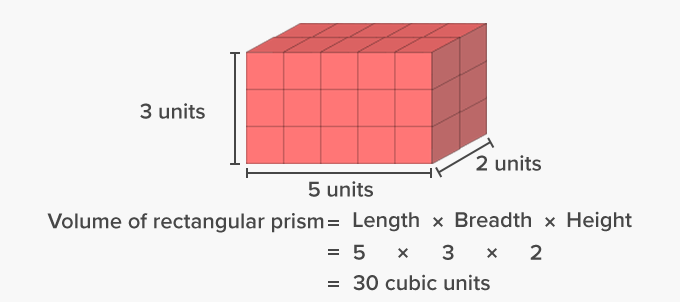Volume of basic solid geometric shapes like cubes and rectangular prisms can be determined using formulas.

Here’s the list of the formulas for determining the volume of 3-D shapes.

 Geometric Shape Name: Geometric Shape: Volume Formula: Cube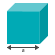Volume = a³  , where a is length of each side. Rectangular Prism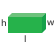Volume = l × w × h , where l is length, w is width and h is height. Sphere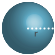Volume = 4/3 πr³ , where r is the radius. Cylinder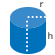Volume = πr²h , where r is the radius and h is the height. Cone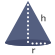Volume = 1/3 πr²h , where r is the radius and h is the height.

 Fun Facts Another way of measuring the volume of an object is to completely submerge the object in water and then, measure the volume of water displaced by the object. The volume of the object is the amount of water displaced.

##Let's do it!

Instead of handing out math worksheets on calculating volume, show how the volume of different figures is measured in different units. Show how to take the overall measurements of a cube, cuboid or sphere-shaped object and then compute its volume. Also, discuss the unit in which the volume will be determined and how it will differ in each case.

##Related math vocabulary

Won Numerous Awards & Honors